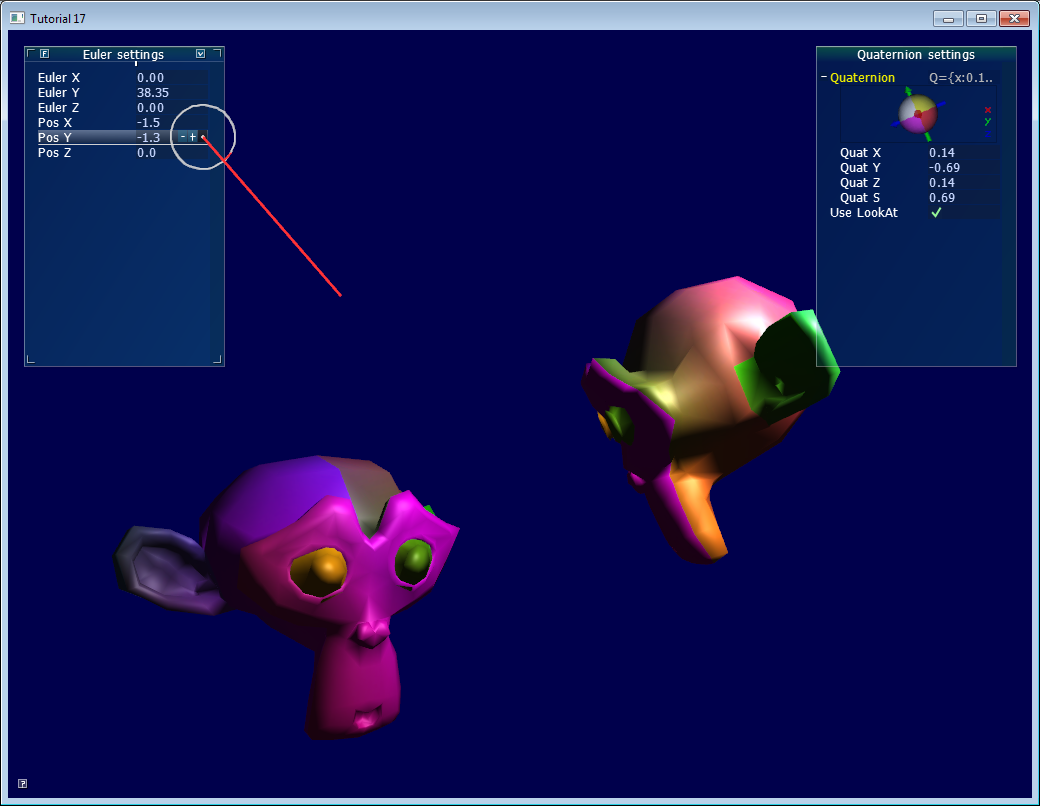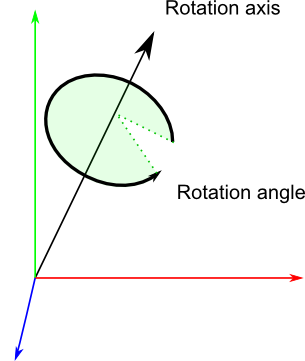This tutorial goes a bit outside the scope of OpenGL, but nevertheless tackles a very common problem: how to represent rotations ?

In Tutorial 3 - Matrices, we learnt that matrices are able to rotate a point around a specific axis. While matrices are a neat way to transform vertices, handling matrices is difficult: for instance, getting the rotation axis from the final matrix is quite tricky.

We will present the two most common ways to represent rotation: Euler angles and Quaternions. Most importantly, we will explain why you should probably use Quaternions.# Foreword: rotation VS orientation

While reading articles on rotations, you might get confused because of the vocabulary. In this tutorial:

• An orientation is a state: “the object’s orientation is…”
• A rotation is an operation: “Apply this rotation to the object”

That is, when you apply a rotation, you change the orientation. Both can be represented with the same tools, which leads to the confusion. Now, let’s get started…

# Euler Angles

Euler angles are the easiest way to think of an orientation. You basically store three rotations around the X, Y and Z axes. It’s a very simple concept to grasp. You can use a vec3 to store it:

``````vec3 EulerAngles( RotationAroundXInRadians, RotationAroundYInRadians, RotationAroundZInRadians);
``````

These 3 rotations are then applied successively, usually in this order: first Y, then Z, then X (but not necessarily). Using a different order yields different results.

One simple use of Euler angles is setting a character’s orientation. Usually game characters do not rotate on X and Z, only on the vertical axis. Therefore, it’s easier to write, understand and maintain “float direction;” than 3 different orientations.

Another good use of Euler angles is an FPS camera: you have one angle for the heading (Y), and one for up/down (X). See common/controls.cpp for an example.

However, when things get more complex, Euler angle will be hard to work with. For instance :

• Interpolating smoothly between 2 orientations is hard. Naively interpolating the X,Y and Z angles will be ugly.
• Applying several rotations is complicated and unprecise: you have to compute the final rotation matrix, and guess the Euler angles from this matrix
• A well-known problem, the “Gimbal Lock”, will sometimes block your rotations, and other singularities which will flip your model upside-down.
• Different angles make the same rotation ( -180° and 180°, for instance )
• It’s a mess - as said above, usually the right order is YZX, but if you also use a library with a different order, you’ll be in trouble.
• Some operations are complicated: for instance, rotation of N degrees around a specific axis.

Quaternions are a tool to represent rotations, which solves these problems.

# Quaternions

A quaternion is a set of 4 numbers, [x y z w], which represents rotations the following way:

``````// RotationAngle is in radians
x = RotationAxis.x * sin(RotationAngle / 2)
y = RotationAxis.y * sin(RotationAngle / 2)
z = RotationAxis.z * sin(RotationAngle / 2)
w = cos(RotationAngle / 2)
``````

RotationAxis is, as its name implies, the axis around which you want to make your rotation.

RotationAngle is the angle of rotation around this axis.So essentially quaternions store a rotation axis and a rotation angle, in a way that makes combining rotations easy.

This format is definitely less intuitive than Euler angles, but it’s still readable: the xyz components match roughly the rotation axis, and w is the acos of the rotation angle (divided by 2). For instance, imagine that you see the following values in the debugger: [ 0.7 0 0 0.7 ]. x=0.7, it’s bigger than y and z, so you know it’s mostly a rotation around the X axis; and 2*acos(0.7) = 1.59 radians, so it’s a rotation of 90°.

Similarly, [0 0 0 1] (w=1) means that angle = 2acos(1) = 0, so this is a *unit quaternion, which makes no rotation at all.

## Basic operations

Knowing the math behind the quaternions is rarely useful: the representation is so unintuitive that you usually only rely on utility functions which do the math for you. If you’re interested, see the math books in the Useful Tools & Links page.

### How do I create a quaternion in C++ ?

``````// Don't forget to #include <glm/gtc/quaternion.hpp> and <glm/gtx/quaternion.hpp>

// Creates an identity quaternion (no rotation)
quat MyQuaternion;

// Direct specification of the 4 components
// You almost never use this directly
MyQuaternion = quat(w,x,y,z);

// Conversion from Euler angles (in radians) to Quaternion
vec3 EulerAngles(90, 45, 0);
MyQuaternion = quat(EulerAngles);

// Conversion from axis-angle
// In GLM the angle must be in degrees here, so convert it.
MyQuaternion = gtx::quaternion::angleAxis(degrees(RotationAngle), RotationAxis);
``````

### How do I create a quaternion in GLSL ?

You don’t. Convert your quaternion to a rotation matrix, and use it in the Model Matrix. Your vertices will be rotated as usual, with the MVP matrix.

In some cases, you might actually want to use quaternions in GLSL, for instance if you do skeletal animation on the GPU. There is no quaternion type in GLSL, but you can pack one in a vec4, and do the math yourself in the shader.

### How do I convert a quaternion to a matrix ?

``````mat4 RotationMatrix = quaternion::toMat4(quaternion);
``````

You can now use it to build your Model matrix as usual:

``````mat4 RotationMatrix = quaternion::toMat4(quaternion);
...
mat4 ModelMatrix = TranslationMatrix * RotationMatrix * ScaleMatrix;
// You can now use ModelMatrix to build the MVP matrix
``````

#

# So, which one should I choose ?

Choosing between Euler angles and quaternions is tricky. Euler angles are intuitive for artists, so if you write some 3D editor, use them. But quaternions are handy for programmers, and faster too, so you should use them in a 3D engine core.

The general consensus is exactly that: use quaternions internally, and expose Euler angles whenever you have some kind of user interface.

You will be able to handle all you will need (or at least, it will be easier), and you can still use Euler angles for entities that require it ( as said above: the camera, humanoids, and that’s pretty much it) with a simple conversion.

# Cheat-sheet

How do I know it two quaternions are similar ?

When using vector, the dot product gives the cosine of the angle between these vectors. If this value is 1, then the vectors are in the same direction.

With quaternions, it’s exactly the same:

``````float matching = quaternion::dot(q1, q2);
if ( abs(matching-1.0) < 0.001 ){
// q1 and q2 are similar
}
``````

You can also get the angle between q1 and q2 by taking the acos() of this dot product.

## How do I apply a rotation to a point ?

You can do the following:

``````rotated_point = orientation_quaternion *  point;
``````

… but if you want to compute your Model Matrix, you should probably convert it to a matrix instead.

Note that the center of rotation is always the origin. If you want to rotate around another point:

``````rotated_point = origin + (orientation_quaternion * (point-origin));
``````

## How do I interpolate between 2 quaternions ?

This is called a SLERP: Spherical Linear intERPolation. With GLM, you can do this with mix:

``````glm::quat interpolatedquat = quaternion::mix(quat1, quat2, 0.5f); // or whatever factor
``````

## How do I cumulate 2 rotations ?

Simple ! Just multiply the two quaternions together. The order is the same as for matrices, i.e. reverse:

``````quat combined_rotation = second_rotation * first_rotation;
``````

## How do I find the rotation between 2 vectors ?

(in other words: the quaternion needed to rotate v1 so that it matches v2)

The basic idea is straightforward:

• The angle between the vectors is simple to find: the dot product gives its cosine.
• The needed axis is also simple to find: it’s the cross product of the two vectors.

The following algorithm does exactly this, but also handles a number of special cases:

``````quat RotationBetweenVectors(vec3 start, vec3 dest){
start = normalize(start);
dest = normalize(dest);

float cosTheta = dot(start, dest);
vec3 rotationAxis;

if (cosTheta < -1 + 0.001f){
// special case when vectors in opposite directions:
// there is no "ideal" rotation axis
// So guess one; any will do as long as it's perpendicular to start
rotationAxis = cross(vec3(0.0f, 0.0f, 1.0f), start);
if (gtx::norm::length2(rotationAxis) < 0.01 ) // bad luck, they were parallel, try again!
rotationAxis = cross(vec3(1.0f, 0.0f, 0.0f), start);

rotationAxis = normalize(rotationAxis);
}

rotationAxis = cross(start, dest);

float s = sqrt( (1+cosTheta)*2 );
float invs = 1 / s;

return quat(
s * 0.5f,
rotationAxis.x * invs,
rotationAxis.y * invs,
rotationAxis.z * invs
);

}
``````

(You can find this function in common/quaternion_utils.cpp)

## I need an equivalent of gluLookAt. How do I orient an object towards a point ?

Use RotationBetweenVectors !

``````// Find the rotation between the front of the object (that we assume towards +Z,
// but this depends on your model) and the desired direction
quat rot1 = RotationBetweenVectors(vec3(0.0f, 0.0f, 1.0f), direction);
``````

Now, you might also want to force your object to be upright:

``````// Recompute desiredUp so that it's perpendicular to the direction
// You can skip that part if you really want to force desiredUp
vec3 right = cross(direction, desiredUp);
desiredUp = cross(right, direction);

// Because of the 1rst rotation, the up is probably completely screwed up.
// Find the rotation between the "up" of the rotated object, and the desired up
vec3 newUp = rot1 * vec3(0.0f, 1.0f, 0.0f);
quat rot2 = RotationBetweenVectors(newUp, desiredUp);
``````

Now, combine them:

``````quat targetOrientation = rot2 * rot1; // remember, in reverse order.
``````

Beware, “direction” is, well, a direction, not the target position ! But you can compute the direction simply: targetPos - currentPos.

Once you have this target orientation, you will probably want to interpolate between startOrientation and targetOrientation.

(You can find this function in common/quaternion_utils.cpp)

## How do I use LookAt, but limit the rotation at a certain speed ?

The basic idea is to do a SLERP ( = use glm::mix ), but play with the interpolation value so that the angle is not bigger than the desired value:

``````float mixFactor = maxAllowedAngle / angleBetweenQuaternions;
quat result = glm::gtc::quaternion::mix(q1, q2, mixFactor);
``````

Here is a more complete implementation, which deals with many special cases. Note that it doesn’t use mix() directly as an optimization.

``````quat RotateTowards(quat q1, quat q2, float maxAngle){

if( maxAngle < 0.001f ){
// No rotation allowed. Prevent dividing by 0 later.
return q1;
}

float cosTheta = dot(q1, q2);

// q1 and q2 are already equal.
// Force q2 just to be sure
if(cosTheta > 0.9999f){
return q2;
}

// Avoid taking the long path around the sphere
if (cosTheta < 0){
q1 = q1*-1.0f;
cosTheta *= -1.0f;
}

float angle = acos(cosTheta);

// If there is only a 2&deg; difference, and we are allowed 5&deg;,
// then we arrived.
if (angle < maxAngle){
return q2;
}

float fT = maxAngle / angle;
angle = maxAngle;

quat res = (sin((1.0f - fT) * angle) * q1 + sin(fT * angle) * q2) / sin(angle);
res = normalize(res);
return res;

}
``````

You can use it like that:

``````CurrentOrientation = RotateTowards(CurrentOrientation, TargetOrientation, 3.14f * deltaTime );
``````

(You can find this function in common/quaternion_utils.cpp)

## How do I…

If you can’t figure it out, drop us an email, and we’ll add it to the list !